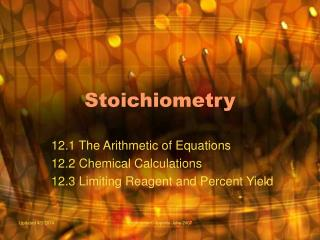DownloadDownload PresentationStoichiometry

# Stoichiometry

Télécharger la présentation## Stoichiometry

- - - - - - - - - - - - - - - - - - - - - - - - - - - E N D - - - - - - - - - - - - - - - - - - - - - - - - - - -
##### Presentation Transcript

1. Stoichiometry 12.1 The Arithmetic of Equations 12.2 Chemical Calculations 12.3 Limiting Reagent and Percent Yield Created by C. Ippolito June 2007

2. The Arithmetic of Equations Objectives Explain how balanced equations apply to both chemistry and everyday life Interpret balanced equations in terms of moles, representative particles, mass, and gas volume at STP Identify the quantities that are always conserved in chemical reactions Created by C. Ippolito June 2007

3. Using Everyday Equations • A balanced chemical equation provides the same kind of quantitative information that a recipe does • Tricycle Recipe Created by C. Ippolito June 2007 3

4. Using Balanced Chemical Equations • Chemists use balanced chemical equations as a basis to calculate how much reactant is needed or product is formed in a reaction • Stoichiometry • study of the calculation of quantities in chemical reactions Created by C. Ippolito June 2007 4

5. Using Balanced Equation as a Recipe Created by C. Ippolito June 2007 5

6. Interpreting Chemical Equations • A balanced equation can represent different quantities: N2(g) + 3H2(g)  2NH3(g) • Atoms • reactants 2 atoms N & 6 atoms H • Molecules • ratio of molecules always 1:3:2 • Moles • coefficients represent moles • Mass • if moles converted to grams • total # of grams of reactants = total # of grams of products • Volume • at STP convert moles to liters (1 mole = 22.4 L) Created by C. Ippolito June 2007 6

7. Mass Conservation in Chemical Reactions • Mass and atoms are conserved Created by C. Ippolito June 2007 7

8. Interpreting a Balanced Chemical Reaction Created by C. Ippolito June 2007 8

9. Chemical Calculations Objectives Construct mole ratios from balanced equations and apply these ratios in stoichiometric calculations Calculate Stoichiometric quantities from balanced chemical equations using units of moles, mass, representative particles and volumes of gases at STP Created by C. Ippolito June 2007

10. Writing and Using Mole Ratios • Mole Ratio • conversion factor derived from coefficients • used to convert between • moles of reactant and moles of products • moles of reactants • moles of products N2(g) + 3H2(g)  2NH3(g) • 3 mole ratios in this equation Created by C. Ippolito June 2007 10

11. Mole-Mole Calculation Created by C. Ippolito June 2007 11

12. Mass-Mass Calculation • Steps to Solve Mass-Mass Problems • change given mass to moles • change moles of given to moles of wanted (unknown) • change moles of wanted to grams Created by C. Ippolito June 2007 12

13. Other Stoichiometric Calculations • In a typical problem: • given converted to moles • moles ratio used to calculate moles of unknown • moles of unknown converted to other unit of measurement Created by C. Ippolito June 2007 13

14. Stoichiometric Calculations Created by C. Ippolito June 2007 14

15. Calculating Molecules of Product Created by C. Ippolito June 2007 15

16. Volume-Volume Calculation Created by C. Ippolito June 2007 16

17. Finding Volume Needed for Reaction Created by C. Ippolito June 2007 17

18. Limiting Reagent and Percent Yield Objectives Identify the limiting reagent in a reaction Calculate theoretical yield, actual yield, or percent yield given appropriate information Created by C. Ippolito June 2007

19. Limiting and Excess Reagents • An insufficient quantity of any of the reactants will limit the amount of product that forms • Limiting Reagent • reactant that determines the amount of product that can be formed in a reaction • Excess Reagent • reactant that is not completely used up in a reaction Created by C. Ippolito June 2007 19

20. Determining Limiting Reagent Created by C. Ippolito June 2007 20

21. Using Limiting Reagent to Find Quantity of Product Created by C. Ippolito June 2007 21

22. Percent Yield • Theoretical Yield • maximum amount of product that could be produced from given amounts of reactants • Actual Yield • amount of product actually formed in the lab • Percent Yield • ratio of the actual yield to the theoretical yield expressed as a percent Created by C. Ippolito June 2007 22

23. Calculating Theoretical Yield Created by C. Ippolito June 2007 23

24. Calculating the Percent Yield Created by C. Ippolito June 2007 24